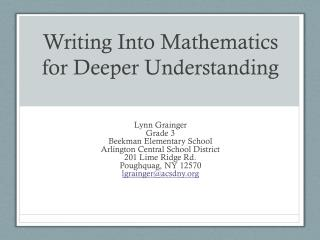# Writing Into Mathematics for Deeper Understanding - PowerPoint PPT PresentationDownload PresentationWriting Into Mathematics for Deeper Understanding

Writing Into Mathematics for Deeper Understanding
Download Presentation## Writing Into Mathematics for Deeper Understanding

- - - - - - - - - - - - - - - - - - - - - - - - - - - E N D - - - - - - - - - - - - - - - - - - - - - - - - - - -
##### Presentation Transcript

1. Writing Into Mathematics for Deeper Understanding Lynn GraingerGrade 3Beekman Elementary SchoolArlington Central School District201 Lime Ridge Rd.Poughquag, NY 12570lgrainger@acsdny.org

2. Question How can writing a word problem based upon a mathematical equation and/or graphic representations enhance a student’s understanding of mathematical concepts?

3. Reasons for Writing in Math Class Support Learning • Examine what they have learned • Reflect on what they have learned Assessment • Emphasis on the process, not just the correct answer • Explain thinking and defend one’s ideas

4. Types of Writing in Math Class Without Revision • Learning Logs • Making Notes • Taking Notes • Think – Write – Share (often on white boards) Published Works • Class Book • Alphabet Book Shared Writing • Teacher recording of student’s understandings with revisions • Classroom Charts

5. Context third grade self-contained heterogeneous class 22 students ranging from ages 7-9 middle class suburban school

6. Literacy Practice • First assignment for you • One traditional word problem with more writing than what is demanded in most math classes • What are the literacy practices for the student in solving this problem?

7. Let’s Talk About It • What literacy skills are being reinforced in the first word problem? • What higher order thinking skills are required?

8. Literacy Practice • Another assignment for you • Let’s look at this in a different way. • What are the literacy practices for the student in solving this problem?

9. Let’s Talk About It • What literacy skills are being reinforced in second and third word problems? • What higher order thinking skills are required? • What does it demand of you? • as a mathematician? • as a reader? • as a writer?

10. What’s the Difference Usually we ask students to decontextualize in order to solve word problems. By providing them with mathematical work we move from the abstract to the concrete. They contextualize the numbers.

11. Supporting Research • Works Cited • Burns, Marilyn. Writing in Math Class: A Resource for Grades 2-8. Sausalito, CA: Math Solutions Publications, 1995. Print. • O'Connell, Susan, Celia Beamon, Susan Denvir, Leila Dowdall, Nikki Friedland, and Joelle Ward. "Aiming for Understanding: Lessons Learned about Writing in Mathematics: Reflect and Discuss." Teaching Children Mathematics (2005): 192. Print. • Parker, Renee, and M. Lynn Breyfogle. "Learning to Write about Mathematics." Teaching Children Mathematics 18.2 (2011): 90. Print. • Staal, Nancy. "Teaching Math All Write." Teaching Children Mathematics 18.4 (2011): 224. Print. • Whitin, Phyllis, and David Whitin. "Promoting Communication in the Mathematics Classroom." Teaching Children Mathematics 9.4 (2001): 205. Print. • Wilcox, Brad, and Eula Ewing Monroe. "Integrating Writing and Mathematics." The Reading Teacher 64.7 (2011): 521-29. Print. • Winograd, K., and K. M. Higgins. "Writing, Reading and Talking Mathematics: One Interdisciplinary Possiblity." Reading Teacher 48.4 (1994): 310-19. Print.

12. Mathematical Practices Focused On In Writing Word Problems • MP1 Make sense of problems and persevere in solving them. • MP2 Reason abstractly and quantitatively. • MP3 Construct viable arguments and critique the reasoning of others. • MP4 Model with mathematics. • MP7 Look for and make use of structure.

13. Critical Areas for Grade 3 • developing understanding of multiplication and division and strategies for multiplication and division within 100; • developing understanding of fractions, especially unit fractions (fractions with numerator 1); • developing understanding of the structure of rectangular arrays and of area; • describing and analyzing two-dimensional shapes

14. Student Work • Let’s talk about what Connor understands. • How does the structure of the assignment help the student?

15. Student Work • With a partner examine the other 3 work samples. • On post-it notes record what student understandings are and what are the student’s needs. • Think about how is the requirement pushing a student’s learning deeper.

16. Back to My Question How can writing a word problem based upon a mathematical equation and/or graphic representations enhance a student’s understanding of mathematical concepts?